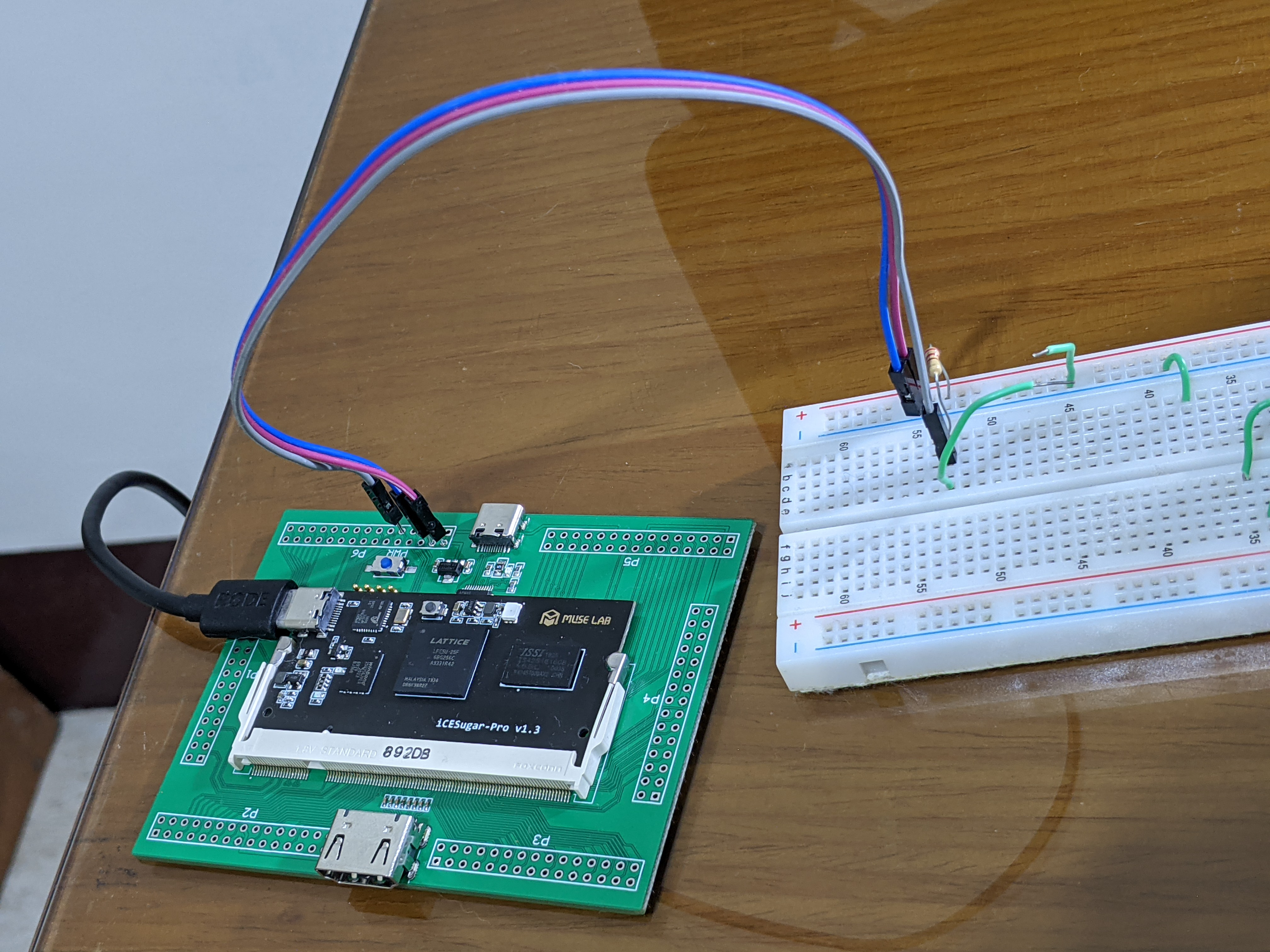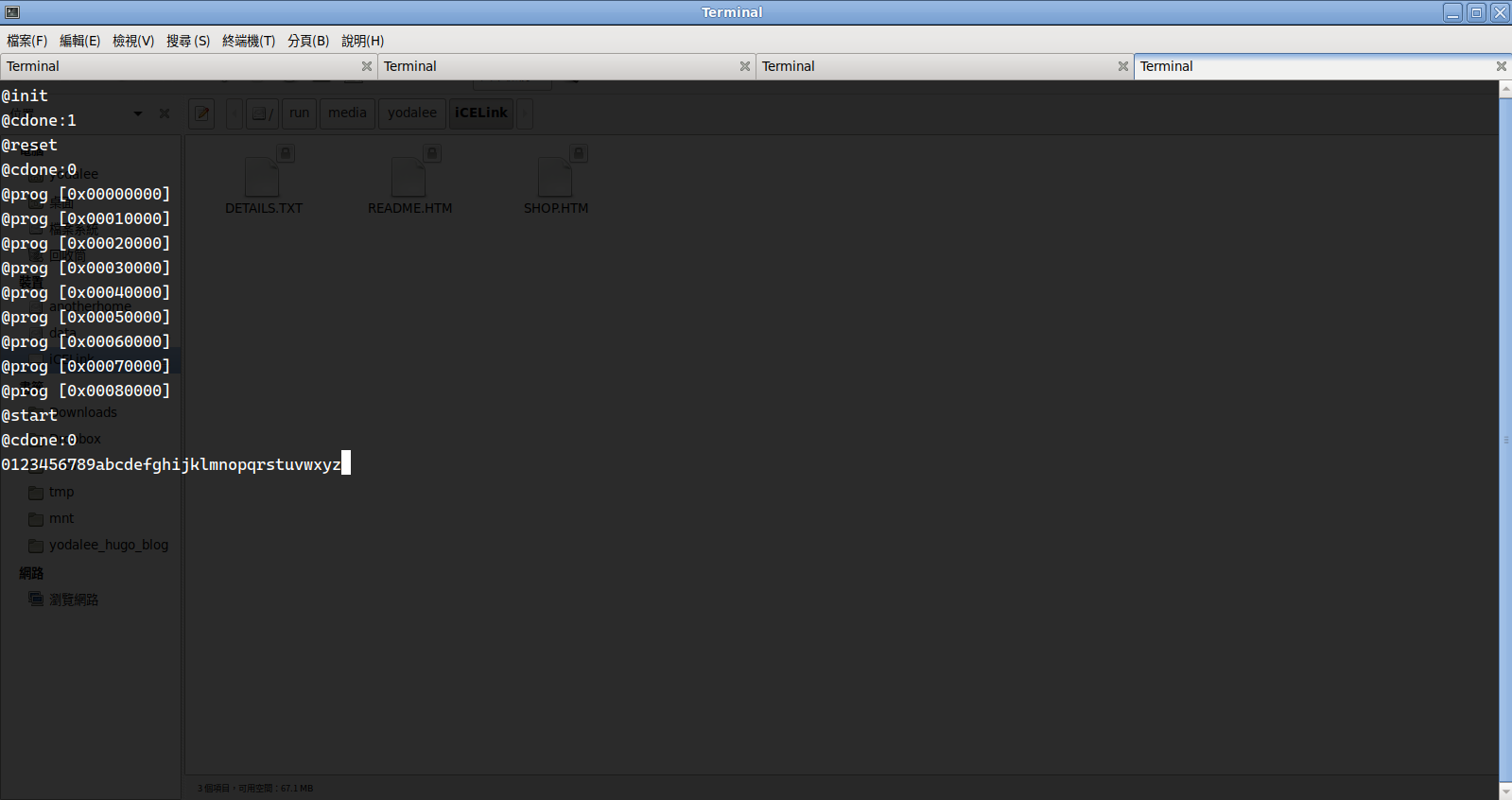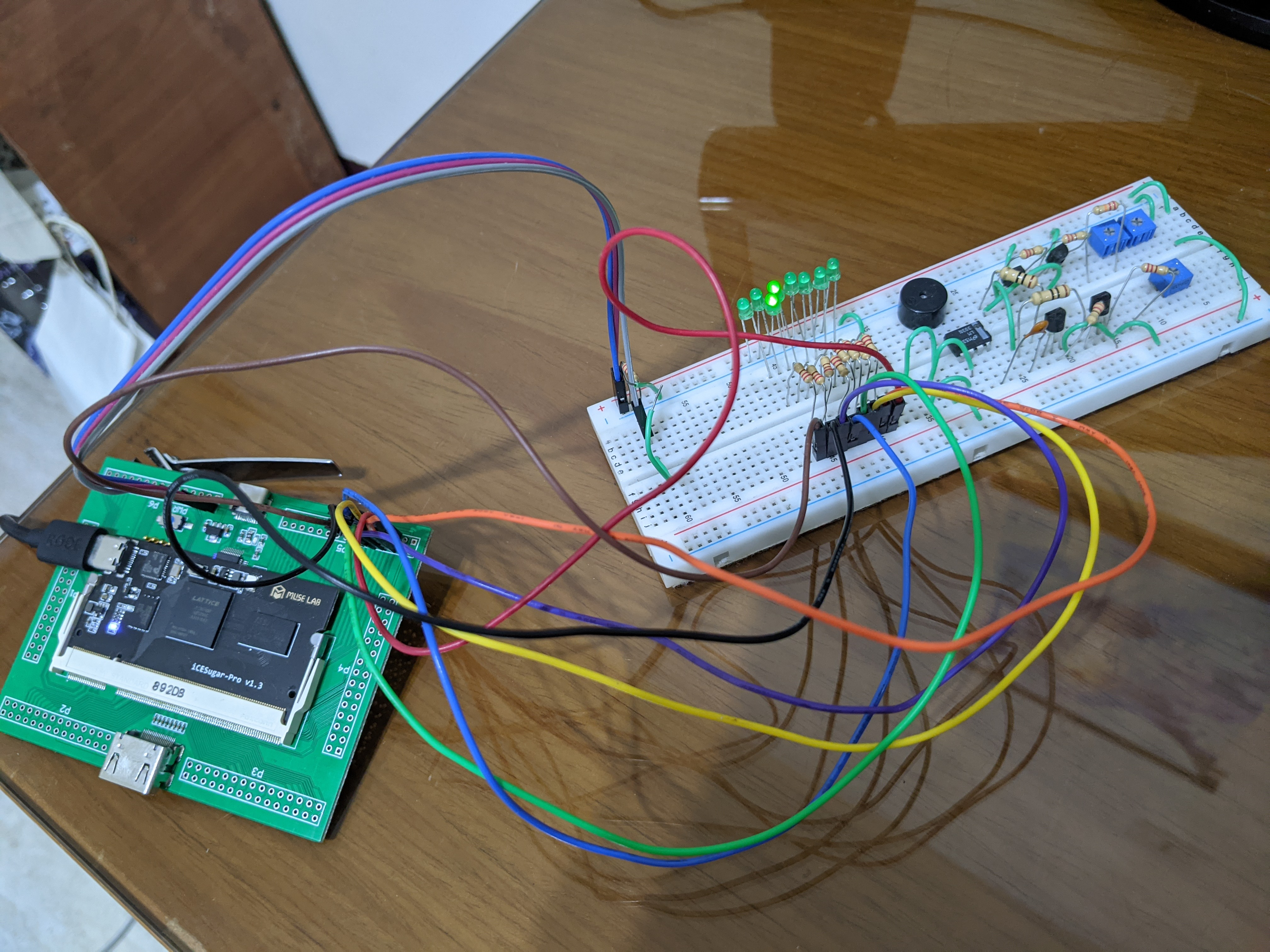icesugar-pro 的 github 上，也附上了一個 UART 的範例 ， 會不斷對你的電腦輸出 “0” 到 “9”（然後這段 code 還有 bug XD），這篇我們就來寫一個有 tx, rx 的 UART 模組吧。

# UART#

UART 全名 Universal Asynchronous Receiver/Transmitter 或譯通用非同步收發傳輸器， 關於它的原理可以參考這篇文章 ， 同一個網站還有提供 VHDL/verilog 的 UART 實作

UART 的相當簡單，靠一根線就能完成傳輸，一般的介面會有 Tx 跟 Rx 兩條線一來一往，未有通訊時通訊線保持在高電位 （這也是古老設備的習慣，有電表示正常，線斷了就會呈現 Open），整個通訊分為四個部分：

1. Idle：維持高電位
2. ST start：從 1->0 表示通訊開始
3. Data 5-9 bits：我對 5-9 bits 這個敘述感到好奇，查了這篇 發現 UART 制定時間早到 1970 年代，那時候的電傳打字機還沒統一，有 5 bits 的 Baudot code ， 7 bits 的 ASCII （是的 ASCII 其實只要 7 bits 就夠了）， 以及最後一統江湖的 byte，成為當今主流 UART 的 8 bits。
4. Parity：可有可無的 Parity bit，可以設定為 odd parity/even parity。
5. Stop bit：1, 1.5 或 2 個 stop bit，直接拉回高電位即可。

# 從 verilog 到 system verilog#

## logic#

verilog 裡面變數分為 wire 跟 reg ，原意是 reg 代表真的有一個實體的暫存器儲存這個值；wire 則是邏輯閘連線的結果，但這兩者在 verilog 裡面很容易混淆，system verilog 用 logic 統一取代；強者我同學 johnjohnlin 大大就開玩笑，從 verilog 轉換到 system verilog 就是：

``````:%s/reg/logic/g
:%s/wire/logic/g
``````

## enum#

system verilog 提供 enum 統一狀態的資料型態，並且可以用 enum 來宣告變數。

## always_ff always_comb always_latch#

verilog 只有一個 always 關鍵字，就有可能因為寫法，合成出 combinational circuit、flip flop、latch 等電路， 就算經驗老道的電路設計師也可能會踩到，合成出不會預期的結果。
system verilog 分割出 always_ff/always_comb/always_latch 三個不同的關鍵字來對應三種合成的電路， 讓合成能進行更多的檢查，確保設計和結果一致。

# 實作#

## reset 信號### LPF 檔#

``````LOCATE COMP "clk" SITE "P6";
IOBUF PORT "clk" IO_TYPE=LVCMOS33;
FREQUENCY PORT "clk" 25 MHZ;

LOCATE COMP "rst" SITE "F14";
IOBUF PORT "rst" IO_TYPE=LVCMOS33;

LOCATE COMP "uart_tx" SITE "B9";
IOBUF PORT "uart_tx" IO_TYPE=LVCMOS33;

LOCATE COMP "uart_rx" SITE "A9";
IOBUF PORT "uart_rx" IO_TYPE=LVCMOS33;
``````
• F14 是 GPIO 的一個 port，可以對照 github 裡面的 接線圖 找到是對應哪個 port。
• B9 是 FPGA 的 UART Tx，在 schematic 上會看到這根線連到 iCElink 的 UART Rx，iCElink 有另一套 UART 透過 USB 接到電腦上。
• 同理 A9 連接到 iCElink UART Tx。

## module UART Tx#

``````module uart_tx (
/* input */
input clk_baud,
input rst,
input [7:0] tx_byte,
input start_send,

/* output */
output logic tx,
output logic done
);

parameter CLK_PER_BAUD = 1;
localparam CLK_CNT_LIMIT = CLK_PER_BAUD-1;
localparam TX_LENGTH = 10; // start 1'b0, 1 byte, end 1'b1

typedef enum logic {
STATE_IDLE  = 0,
STATE_TXING = 1
} State_t;
State_t state = STATE_IDLE, state_next;
logic [9:0] tx_buf;
logic [3:0] tx_idx, tx_idx_next;
int  clk_cnt, clk_cnt_next;
``````

parameter module 是這次新學會的招式， 因為 FPGA 運作頻率遠比 baud rate 9.6 kHz 高很多，由外部生一個 9.6 kHz 的時脈給 UART module 用顯然不合理； 這裡 UART module 會吃全速的 clk，外面用 parameter 告訴 UART module 這個時脈是多少。

``````/* update state logic */
always_ff @(posedge clk or negedge rst) begin
if (!rst) begin
state   <= STATE_IDLE;
tx_buf  <= 0;
tx_cnt  <= 0;
clk_cnt <= 0;
end
else begin
if (state == STATE_IDLE && start_send) begin
tx_buf <= {1'b1, tx_byte, 1'b0};
end
state   <= state_next;
tx_cnt  <= tx_cnt_next;
clk_cnt <= clk_cnt_next;
end
end
``````

tx_idx 記錄現在要送第幾個位元；clk_cnt 計算 clk 以產生符合 9600 kHz 的時脈。

``````/* next logic for clk_cnt */
always_comb begin
clk_cnt_next = (state == STATE_IDLE || clk_cnt == CLK_CNT_LIMIT) ? 0 : clk_cnt+1;
end

/* next logic for state */
always_comb begin
case (state)
STATE_IDLE: begin
state_next = (start_send == 1'b1) ? STATE_TXING : state;
end
STATE_TXING: begin
if (clk_cnt == CLK_CNT_LIMIT && tx_idx == TX_LENGTH-1) begin
state_next = STATE_IDLE;
end
else begin
state_next = state; end
end
endcase
end
``````

output 邏輯的部分就沒什麼特別的了。

``````/* output logic */
assign done = tx_idx == TX_LENGTH - 1;
assign tx = (state == STATE_IDLE) ? 1'b1 : tx_buf[tx_idx];
``````

## module UART Rx#

rx 模組其實跟 tx 沒差很多：

``````module uart_rx (
/* input */
input clk,
input rst,
input rx,

/* output */
output logic [7:0] rx_byte,
output logic done
);

parameter CLK_PER_BAUD = 1;
localparam CLK_CNT_LIMIT = CLK_PER_BAUD-1;
localparam SAMPLE_CLK_CNT = CLK_CNT_LIMIT / 2;
localparam RX_LENGTH = 10; // start 1'b0, 1 byte, end 1'b1

typedef enum logic {
STATE_IDLE  = 0,
STATE_RXING = 1
} State_t;
State_t state = STATE_IDLE, state_next;
logic [9:0] rx_buf;
logic [3:0] rx_idx, rx_idx_next;
int  clk_cnt, clk_cnt_next;

/* output logic */
assign done = rx_idx == RX_LENGTH - 1;
assign rx_byte = rx_buf[8:1];
``````

``````/* next logic for clk_cnt */
always_comb begin
clk_cnt_next = (state == STATE_IDLE || clk_cnt == CLK_CNT_LIMIT) ? 0 : clk_cnt+1;
end

/* next logic for state */
always_comb begin
case (state)
STATE_IDLE: begin
state_next = (rx == 1'b0) ? STATE_RXING : state;
end
STATE_RXING: begin
if (clk_cnt == CLK_CNT_LIMIT && rx_idx == RX_LENGTH-1) begin state_next = STATE_IDLE; end
else begin state_next = state; end
end
endcase
end

/* next logic for rx_idx */
always_comb begin
if (state == STATE_RXING && rx_idx < RX_LENGTH) begin
if (clk_cnt == CLK_CNT_LIMIT) begin
rx_idx_next = rx_idx+1;
end
else begin
rx_idx_next = rx_idx;
end
end
else begin
rx_idx_next = 0;
end
end

/* update state logic */
always_ff @(posedge clk or negedge rst) begin
if (!rst) begin
state   <= STATE_IDLE;
rx_buf  <= 0;
rx_idx  <= 0;
clk_cnt <= 0;
end
else begin
if (clk_cnt == SAMPLE_CLK_CNT) begin
rx_buf[rx_idx] <= rx;
end
state   <= state_next;
rx_idx  <= rx_idx_next;
clk_cnt <= clk_cnt_next;
end
end
``````

## module Top#

``````module top (
input clk,
input rst,
input  rx,
output tx,
);

parameter CLK_FREQ = 25_000_000;
parameter BAUDRATE = 9600;
parameter CLK_PER_BAUD = CLK_FREQ / BAUDRATE;

byte rx_byte;
logic uart_rxed;
``````

top module 對外連結 UART 的 tx, rx 線，用 parameter 算出 CLK_PER_BAUD，如果要修改 baud rate，改寫這裡即可

``````uart_tx # (.CLK_PER_BAUD(CLK_PER_BAUD))
mod_uart_tx (
/* input */
.clk(clk),
.rst(rst),
.tx_byte(rx_byte),
.start_send(uart_rxed),

/* output */
.tx(tx),
.done(uart_txed)
);

uart_rx # (.CLK_PER_BAUD(CLK_PER_BAUD))
mod_uart_rx (
/* input */
.clk(clk),
.rst(rst),
.rx(rx),

/* output */
.rx_byte(rx_byte),
.done(uart_rxed)
);
``````

## 測試#

icesugar 會透過 iCElink，一次把可用的介面都打開，UART 介面會開在 /dev/ttyACM0，可以用 `screen /dev/ttyACM0 -s 9600` 來與我們的 UART 模組溝通，如果你對付的是其他設定的 UART protocol，可以透過 stty 設定 tty 裝置的參數